Function Repository Resource:

# SchurS

Evaluate the Schur polynomial corresponding to an integer partition

Contributed by: Jan Mangaldan
 ResourceFunction["SchurS"][p,{x1,…,xn}] gives the Schur polynomial sp(x1,…,xn) corresponding to the integer partition p in the variables x1,…,xn.

## Details

Schur polynomials are also called Schur functions.
The Schur polynomial is defined as the sum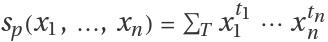, where T ranges over all the semi-standard Young tableaux with shape corresponding to the integer partition p, and the exponents tj count the number of occurences of the number j in the semi-standard Young tableau T.
The Schur polynomials are the characters of polynomial irreducible representations of the general linear groups in representation theory.
The Schur polynomial is a symmetric polynomial of n variables, and is thus invariant under any permutation of its variables.
The integer partition p should be a list of weakly decreasing non-negative integers.
The degree of ResourceFunction["SchurS"][p,{x1,,xn}] is equal to Total[p].
The Schur polynomials form a basis for the symmetric polynomials.

## Examples

### Basic Examples (1)

A Schur polynomial in two variables:

 In:=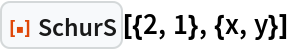Out=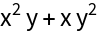### Scope (2)

Generate all partitions of 8 into at most 3 integers:

 In:=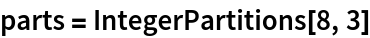Out=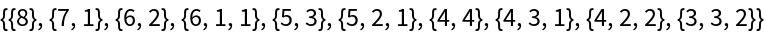Generate all Schur polynomials in three variables of degree 8:

 In:=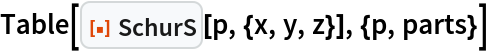Out=### Applications (4)

Count the number of semi-standard Young tableaux of shape (4 2 1 1), with entries taken from the numbers 1 to 4:

 In:=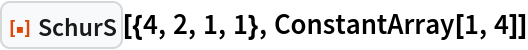Out=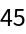Verify Jacobi's bialternant formula for the Schur polynomial:

 In:=Out=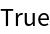Generate all monomial symmetric polynomials of degree 5:

 In:=Out=Generate the Kostka numbers as the coefficients that arise when the Schur polynomial is expanded in the monomial symmetric basis:

 In:=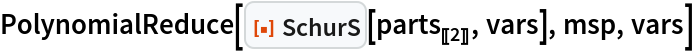Out=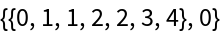The Littlewood-Richardson rule states that the product of two Schur polynomials can be expressed as a linear combination of Schur polynomials with integer coefficients. Generate the product from the original example by Littlewood and Richardson:

 In:=Out=Use PolynomialReduce to find the coefficients for the Schur polynomial terms of the product (this might take a while to evaluate):

 In:=Out=Show the mapping between the nonzero coefficients and their corresponding partitions:

 In:=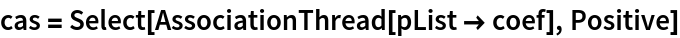Out=Reconstruct the original polynomial:

 In:=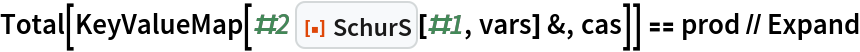Out=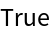### Properties and Relations (1)

PolynomialReduce can be used to find the coefficients for the expansion of any symmetric polynomial in the Schur basis:

 In:=Out=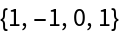## Version History

• 1.0.0 – 10 April 2023